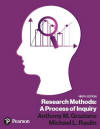﻿ Nature of StatisticsGraziano & Raulin
Research Methods (9th edition)

## Nature of Statistics

The Graziano and Raulin textbook covered the basics of statistical procedures, but this section will review those critical concepts and expand on them.

### Purpose of Statistics

Statistics serve two primary purposes. The first is to summarize and describe a set of data from a research study. The second is to provide an objective basis for drawing conclusions from the data collected in a research study.

### Key Definitions

Listed here are definitions of critical terms for this section.

• descriptive statistics - statistics that are used to describe some aspect of the data, such as the typical score or the variability of scores.

• inferential statistics - statistical procedures that are used to draw conclusions about the populations under study based on the sample data from those populations.

• population - all people, objects, or events that are of interest. Populations are usually defined by one or more specific characteristics. For example, we might be studying the population of all college students, which is defined as all individuals currently attending college.

• sample - a subset of people, objects, or events drawn from the population. The word sample is also used as a verb to indicate the process of drawing the sample.

• population parameter - a descriptive statistic that is computed on the entire population.

• sample statistic - a descriptive statistic that is computed on a sample drawn from the population.

• sampling procedure - the method that is used to draw a sample from the population.

• sample size - the number of individuals included in the sample that is drawn from the population. Traditionally, the uppercase letter N is used to denote this number.

### Sampling Procedure

The object of sampling is to obtain a sample that is representative of the population from which the sample is drawn. A sample is representative of the population if it shows the same characteristics as the population. In other words, the sample statistics are very similar to the population parameters.

The best way of obtaining a representative sample is to sample randomly, which means sampling so that every individual in the population has an equal chance of being sampled and the selection of any one individual does not change the probability of sampling any other individual. As you will learn in the research methods textbook, random sampling is very difficult to actually carry out, and research rarely is based on true random samples from the population.

One can think of statistical procedures as a way of drawing conclusions about population parameters based on sample statistics. As you will learn later, sample statistics computed on large samples tend to provide more precise estimates of population parameters than statistics computed on small samples. Therefore, using larger samples will increase our sensitivity to the characteristics of the population, which is a concept that we will be discussing in more detail later.